# A Natural GCD Algorithm

The greatest common divisor (gcd) of two non-negative integers is the largest integer that divides both of them, so for example

```gcd 15 6 == 3 gcd 21 10 == 1 gcd 10 0 == 10 gcd 0 0 == 0  -- by convention```

Two numbers that have a gcd of 1 (like 21 and 10) are said to be coprime. Although in general it’s a hard problem to factor an integer into divisors

```91 == 7 * 13 69 == 3 * 13```

it’s an easy problem to compute the gcd of two integers

`gcd 91 69 == 13`

and if they are not coprime then a non-trivial divisor of both is revealed.

Here is a simple recursive definition that efficiently computes gcd:

```gcd :: Integer -> Integer -> Integer gcd a 0 = a gcd a b = gcd b (a `mod` b)```

How would we check that this definition always computes the gcd of two non-negative integers? If we define a divides relation

```divides :: Integer -> Integer -> Bool divides 0 b = b == 0 divides a b = b `mod` a == 0 ```

then we can easily test that gcd returns some common divisor:

```commonDivisorTest :: Integer -> Integer -> Bool commonDivisorTest a b = let g = gcd a b in divides g a && divides g b```

But how to check that gcd returns the greatest common divisor? Consider the linear combination g of a and b

`g = s * a + t * b`

where the coefficients s and t are arbitrary integers. Any common divisor of a and b must also be a divisor of g, and so if g is itself a common divisor of a and b then it must be the greatest common divisor. The extended gcd algorithm computes the coefficients s and t together with the gcd:

```egcd :: Integer -> Integer -> (Integer,(Integer,Integer)) egcd a 0 = (a,(1,0)) egcd a b =     (g, (t, s - (a `div` b) * t))   where     (g,(s,t)) = egcd b (a `mod` b)```

which allows us to test that egcd returns the greatest common divisor:

```greatestCommonDivisorTest :: Integer -> Integer -> Bool greatestCommonDivisorTest a b =     let (g,(s,t)) = egcd a b in     divides g a && divides g b && s * a + t * b == g```

The coefficients s and t are useful for much more than checking the gcd result: for any coprime a and b we have

```s * a + t * b == 1 (s * a) `mod` b == 1  -- assuming b > 1 (t * b) `mod` a == 1  -- assuming a > 1```

which shows that s is the multiplicative inverse of a modulo b, and t is the multiplicative inverse of b modulo a.

### Computing Over the Natural Numbers

The extended gcd algorithm is a classic integer algorithm, but what if we wanted a version that computed over the type of natural numbers? This would be useful in environments in which unsigned arithmetic is more natural and/or efficient than signed arithmetic (one such environment being the natural number theory of an interactive theorem prover, which is where this need arose).

The immediate problem is that one of the s and t coefficients returned by the extended gcd algorithm is usually a negative number, which is to be expected since they must satisfy

`s * a + t * b == g`

where g is generally less than both a and b. The solution is to modify the specification and ask for natural number coefficients s and t that satisfy

`s * a == t * b + g`

Fortunately for us, it turns out that such coefficients always exist except when a is zero (and b is non-zero).

Unfortunately for us, our modified specification is no longer symmetric in a and b. Given coprime a and b as input, s is the multiplicative inverse of a modulo b as before, but t is now the negation of the multiplicative inverse of b modulo a (the additive inverse of the multiplicative inverse—funny). This asymmetry breaks the recursion step of the extended gcd algorithm, because there is no longer a way to derive coefficients s and t satisfying

`s * a == t * b + g`

from coefficients s’ and t’ satisfying

`s' * b == t' * (a `mod` b) + g`

[If you try you’ll discover that a and b are the wrong way around.] This problem is solved by unwinding the recursion so that the algorithm makes two reductions before calling itself recursively, giving coefficients s’ and t’ satisfying

`s' * (a `mod` b) == t' * (b `mod` (a `mod` b)) + g`

Although this is expression is more complicated, it is now possible to define coefficients

```s = s' + (b `div` (a `mod` b)) * t' t = t' + (a `div` b) * s```

which satisfy the desired equation

`s * a == t * b + g`

Here is the finished implementation:

```egcd :: Natural -> Natural -> (Natural,(Natural,Natural)) egcd a 0 = (a,(1,0)) egcd a b =     if c == 0     then (b, (1, a `div` b - 1))     else (g, (u, t + (a `div` b) * u))   where     c = a `mod` b     (g,(s,t)) = egcd c (b `mod` c)     u = s + (b `div` c) * t```

This satisfies a modified test confirming that it produces a greatest common divisor (for non-zero a):

```greatestCommonDivisorTest :: Natural -> Natural -> Bool greatestCommonDivisorTest a b =     let (g,(s,t)) = egcd a b in     divides g a && divides g b && s * a == t * b + g```

And we can also check the upper bounds on the s and t coefficients (for non-zero a and b at least 2):

```coefficientBoundTest :: Natural -> Natural -> Bool coefficientBoundTest a b =     let (_,(s,t)) = egcd a b in     s < b && t < a```

### Application: The Chinese Remainder Theorem

The Chinese Remainder Theorem states that for coprime a and b, given an x and y satisfying

```x < a y < b```

there is a unique n satisfying

```n < a * b n `mod` a == x n `mod` b == y```

But how to compute this n given natural number inputs a, b, x and y? We build on the natural number extended gcd algorithm, of course:

```chineseRemainder :: Natural -> Natural -> Natural -> Natural -> Natural chineseRemainder a b =     \x y -> (x * tb + y * sa) `mod` ab   where     (_,(s,t)) = egcd a b     ab = a * b     sa = s * a     tb = (a - t) * b```

The λ expression in the body maximizes the precomputation on a and b, allowing efficient Chinese remaindering of different x and y inputs once the a and b inputs have been fixed. As with all computation over the natural numbers we must be careful with the subtraction operation `(a - t)` to ensure that the first argument is always larger than the second, but here we know from the `coefficientBoundTest` that t is always less than a.

### References

All of the natural number computations in this blog post have been formally verified and are available as the Haskell package opentheory-divides.

Advertisements

# Large Knight’s Tours

I was at middle school when I first heard about the knight’s tour problem: place a knight on any square on a chess board, and move it to every square on the board without visiting any square more than once. A chess knight moves by jumping two squares forward and one square sideways, so a big part of the challenge is getting into and out of all the corners. I remember filling up pages and pages of squared notebooks with attempted solutions, although my record from that time was a tour that covered only 61 of the 64 squares.

### Enter the Computer

Well, it’s one thing to look for knight’s tours by hand, but quite another to search for them with a computer. It is actually a fun programming exercise to search for knight’s tours: it is possible to use a standard backtracking algorithm, but it needs a couple of tricks to make it work. Firstly, if you have a choice of squares to jump to, then you should consider them in ascending order of accessibility (i.e., from how many unvisited squares could you jump to this square). This helps to ensure that you don’t waste last chances to visit squares. However, even with this trick, it is possible to make mistakes early on in the search that you can never recover from, and end up lost forever in a maze with 1051 turns and no exits. An ugly way to fix this is to assign a move budget—I chose 100 times the number of squares—and if you don’t find a solution within this many jumps then give up and start again from an empty board.

Armed with a fast computer, a search algorithm and a couple of tricks, I was finally able to realize a childhood dream and find a knight’s tour:Note that we always begin our tours in the bottom right corner, so that the search program only has to work out how to get into and out of 3 corners: that time spent filling squared notebooks taught me something!

### Building Up

Once the initial problem is solved, I naturally start wondering whether we can find knight’s tours on larger boards. It is easy to modify the search program to change the board dimensions from an 8×8 square to an m×n rectangle, but as the board size increases the time it takes to find a knight’s tour grows rapidly:

 Board size (n×n) 8 10 12 14 16 18 20 22 24 26 28 30 Search time (ms) 1 3 7 11 26 51 102 225 401 701 1,074 1,738

Extrapolating these results to a 100×100 board, it seems that my search program would take over a thousand years to find a knight’s tour! Clearly I need a new approach to find knight’s tours for large boards.

Our 8×8 knight’s tour above looks like a little circuit, and drawn the same way a 100×100 knight’s tour would look like a huge circuit. But let’s take some inspiration from the way that huge circuits are designed in practice: not all at once, but rather by wiring together smaller circuits. Consider this special 6×6 knight’s tour:One reason this knight’s tour is special is because it has no start and end: it is a loop! There are probably clever ways to find knight’s tours that are loops, but I took a simple-minded approach. I modified my program to keep searching for knight’s tours until it found one where the end was a knight’s jump away from the start, and then I connected the ends of the tour to make a loop.

There is another reason that this knight’s tour is special, which is not so easy to see. You can put two of them together: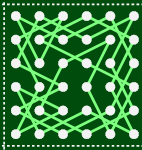then switch two of the jumps around to connect the tours together:and the result is a knight’s tour on a 12×6 board. To see that the result is indeed a tour, let’s visualize the two separate knight’s tours as loops using a schematic diagram: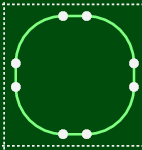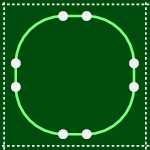And now when we perform the connection step the schematic diagram makes it clear that we have created one big loop from the two separate loops: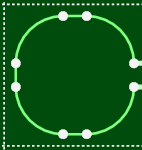The same thing also works by vertically stacking the special 6×6 knight’s tour: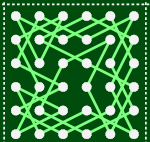and connecting them like so: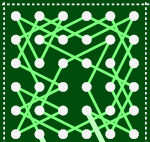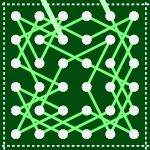### Assembling Large Tours

I’ve shown how to connect special 6×6 knight’s tours together, and the next step is to connect them in an m×n grid to make a knight’s tour of a 6m×6n board. Connecting them together in a grid requires a little bit of care to avoid fragmenting the knight’s tour into disconnected paths. Here’s a schematic diagram for a 2×2 grid illustrating how tours can be fragmented into disconnected paths: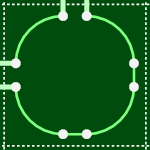One way to avoid tour fragmentation is to connect along some path through the grid that visits every 6×6 knight’s tour. I chose a spiral, starting at the bottom right of the grid, travelling anti-clockwise and ending in the middle. Here is the schematic diagram for a spiral path on a 3×3 grid: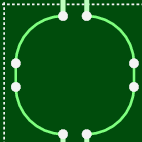And here’s the resulting knight’s tour on an 18×18 board: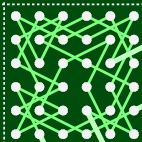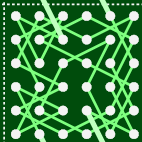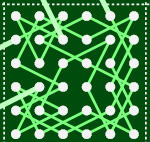### Filling in the Gaps

At this stage we have the machinery to construct knight’s tours for arbitrarily large boards of dimension 6m×6n. But what about boards of other dimensions, such as the 100×100 board that we set as our target? These boards can be handled by searching for more special tours of dimensions m×n, where 6 ≤ m,n ≤ 11, and using these in place of 6×6 knight’s tours on the bottom and right edges of the grid. For example, here is a knight’s tour of a 23×23 board: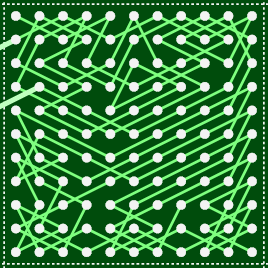This has the same 3×3 grid pattern as the 18×18 knight’s tour, but with larger pieces around the right and bottom edges. Also, the knight’s tour in the bottom right corner is not a loop—you can see one of its ends in the bottom right corner—so the schematic diagram for the assembled tour ends up looking a little different:In fact, it is impossible to find a knight’s tour that is a loop on this board, because it has an odd number of squares. To see why, imagine the squares of the board painted light and dark colors in a checkerboard pattern:As a knight jumps around the board it alternates between light and dark squares, and a tour of a board with an odd number of squares will contain an even number of jumps. This means the start and end squares of the tour will have the same color, and so there’s no way to jump from the end square of the tour back to the start square to close the loop.

### Summary

Using these techniques you can easily assemble knight’s tours for large boards: you can view a tour of a 100×100 board or even assemble your own knight’s tour.

But these techniques only work for boards where the width and height are at least 6: there are also an infinite number of thin boards that might contain knight’s tours:Can you think of techniques for assembling knight’s tours on thin boards? ♘

### Appendix

The performance results were gathered using a MacBook Air with a 1.8GHz Intel Core i7 processor and 4Gb of RAM, running OS X 10.7.5.